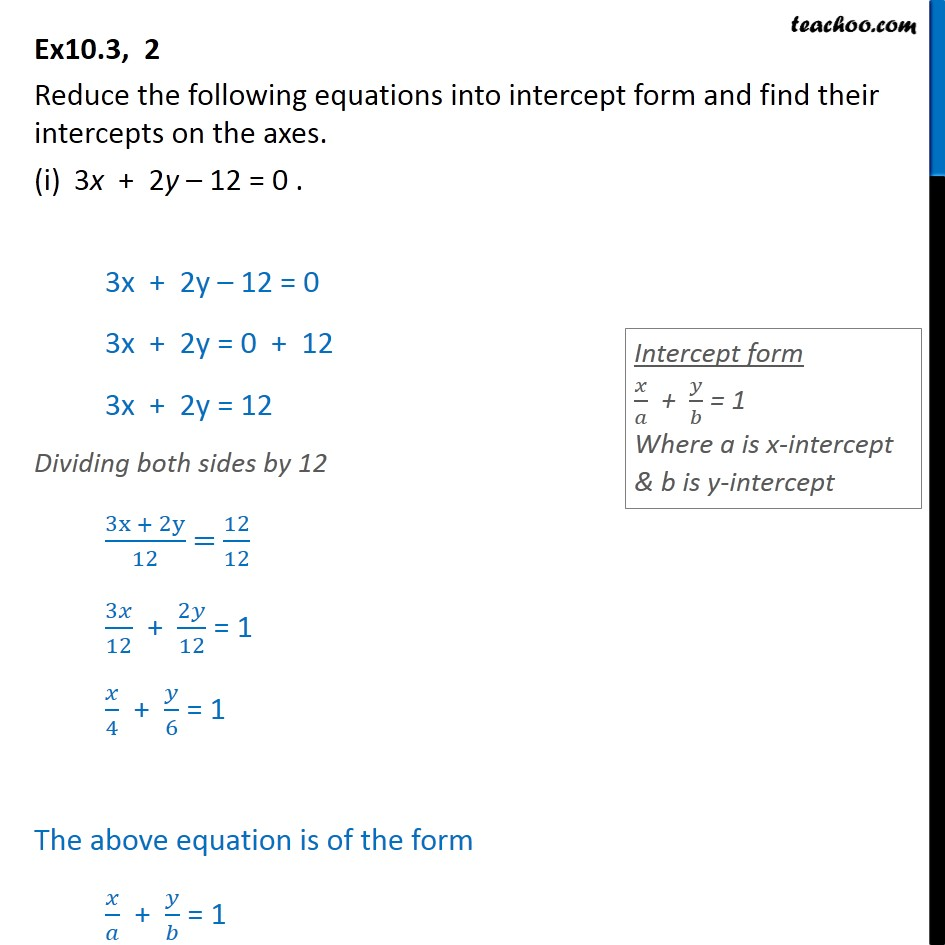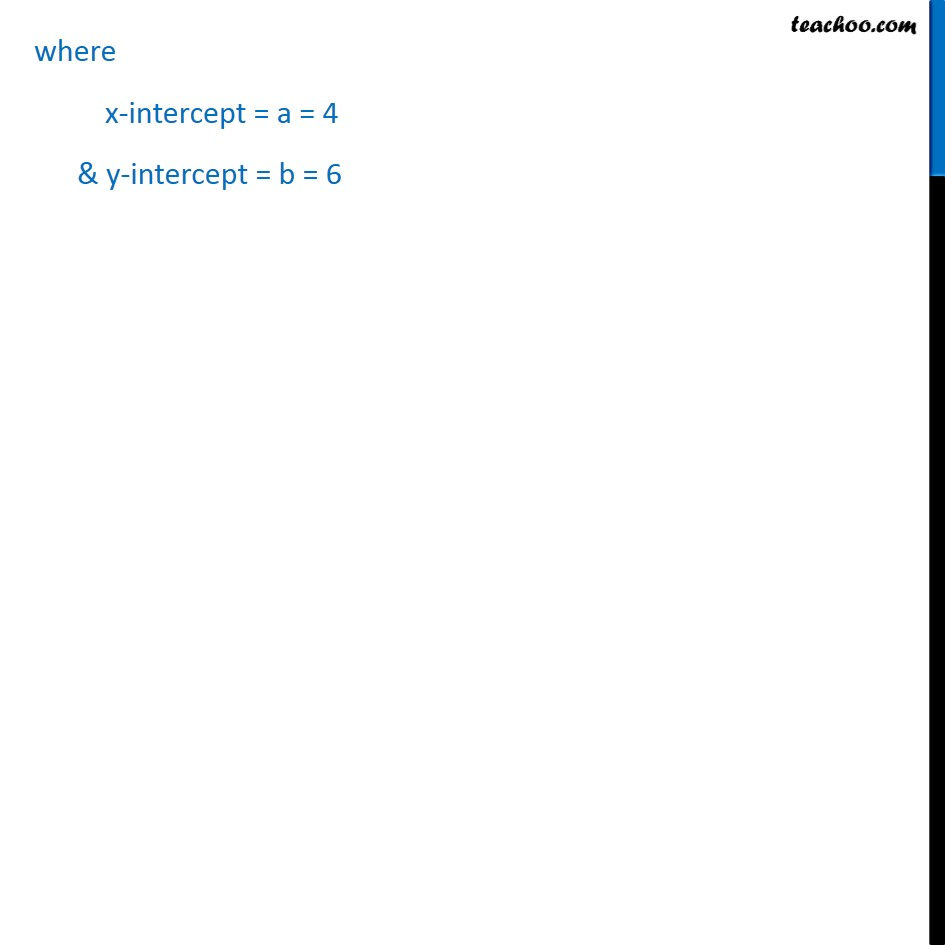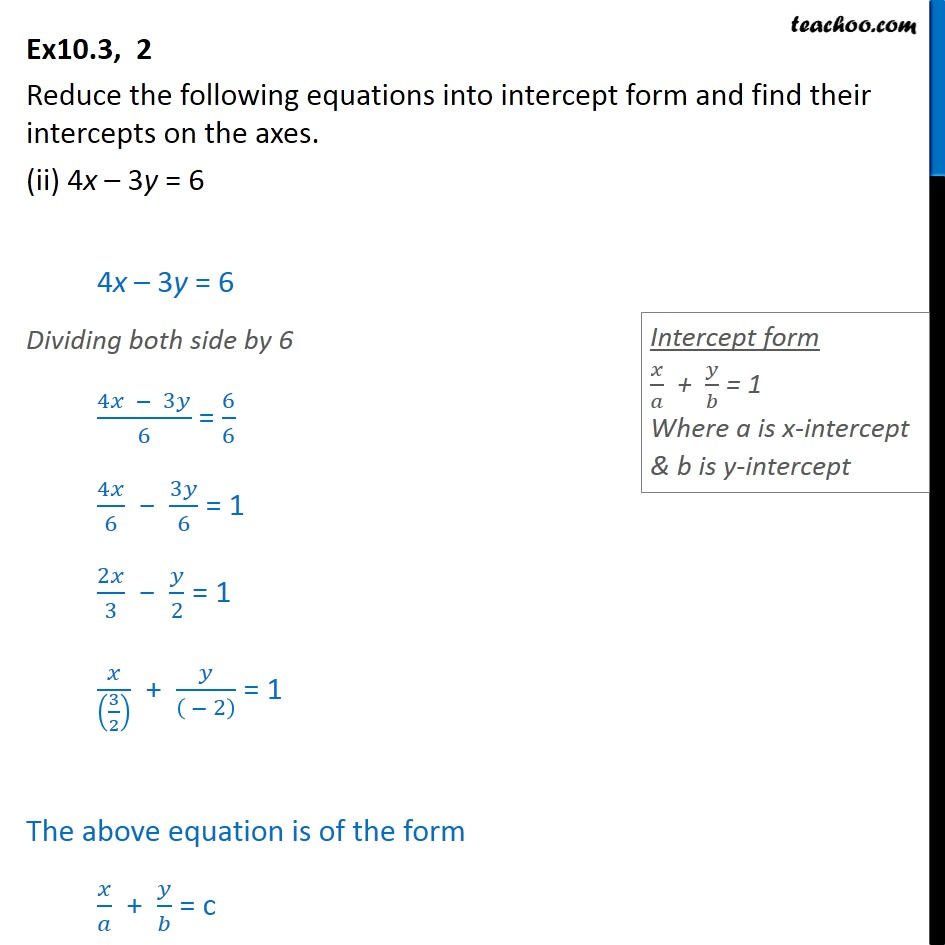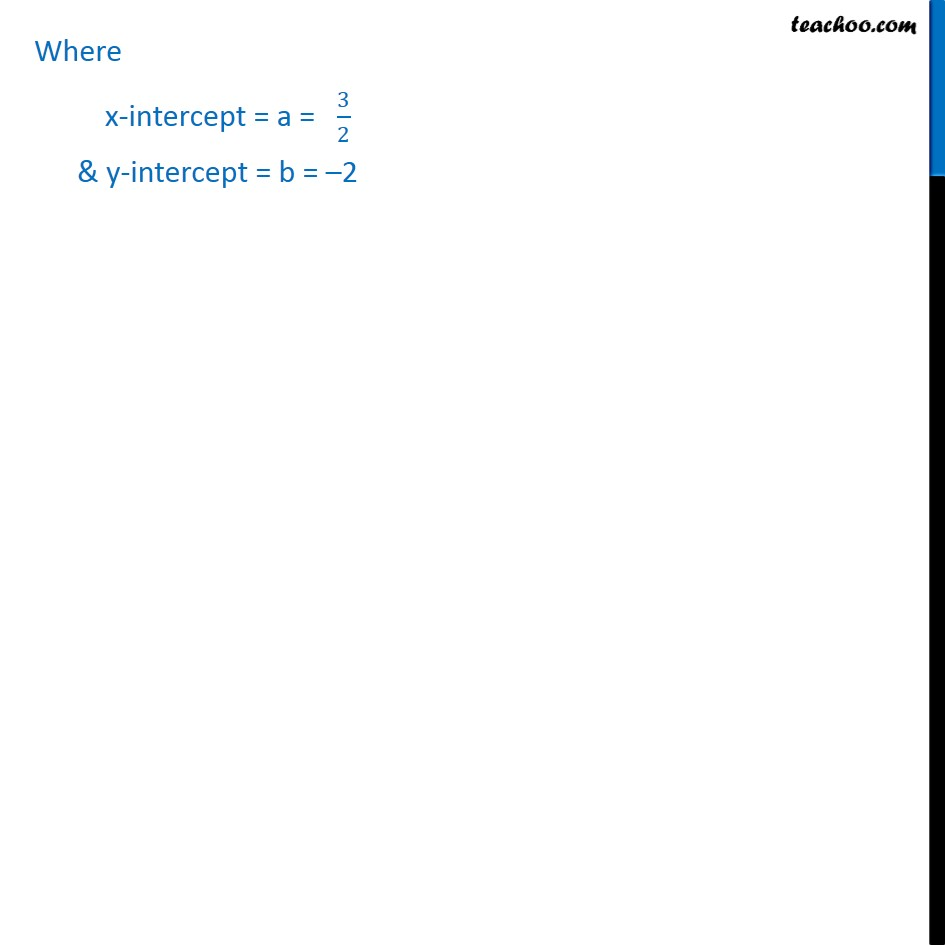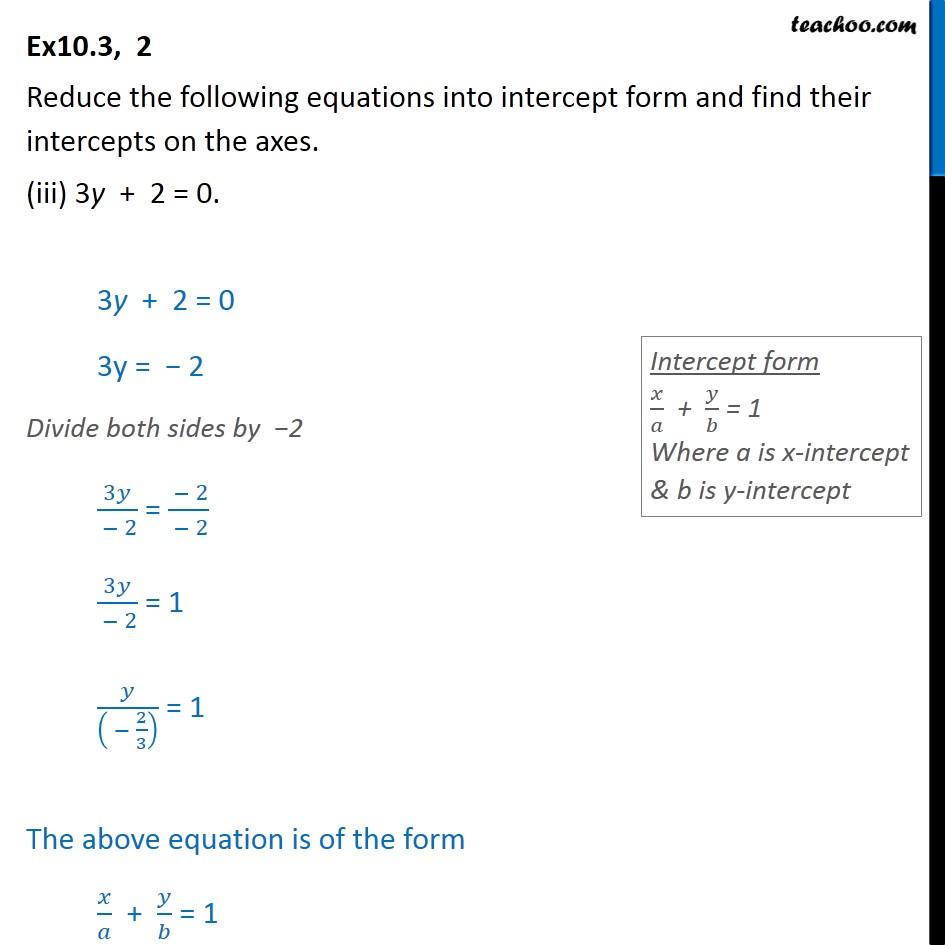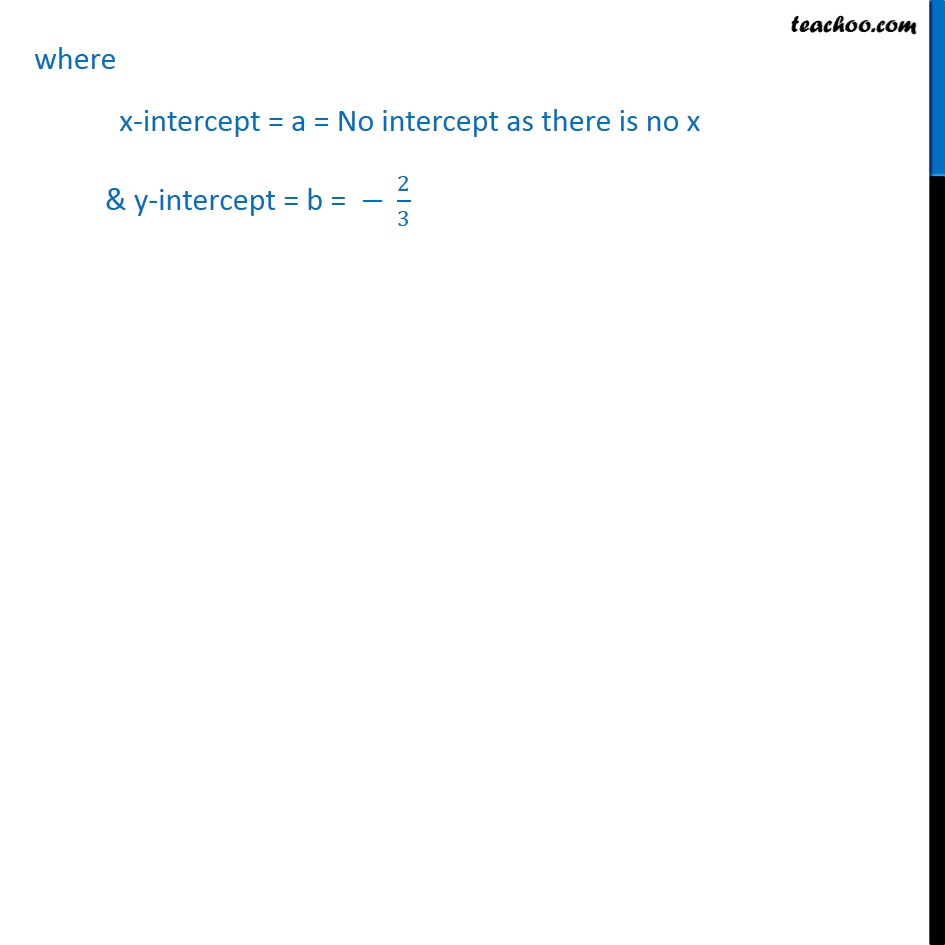Subscribe to our Youtube Channel - https://you.tube/teachoo

1. Chapter 10 Class 11 Straight Lines
2. Serial order wise
3. Ex 10.3

Transcript

Ex10.3, 2 Reduce the following equations into intercept form and find their intercepts on the axes. 3x + 2y 12 = 0 . 3x + 2y 12 = 0 3x + 2y = 0 + 12 3x + 2y = 12 Dividing both sides by 12 (3x + 2y)/12 = 12/12 3 /12 + 2 /12 = 1 /4 + /6 = 1 The above equation is of the form / + / = 1 where x-intercept = a = 4 & y-intercept = b = 6 Ex10.3, 2 Reduce the following equations into intercept form and find their intercepts on the axes. (ii) 4x 3y = 6 4x 3y = 6 Dividing both side by 6 (4 3 )/6 = 6/6 4 /6 3 /6 = 1 2 /3 /2 = 1 /((3/2) ) + /(( 2) ) = 1 The above equation is of the form / + / = c Where x-intercept = a = 3/2 & y-intercept = b = 2 Ex10.3, 2 Reduce the following equations into intercept form and find their intercepts on the axes. (iii) 3y + 2 = 0. 3y + 2 = 0 3y = 2 Divide both sides by 2 3 /( 2) = ( 2)/( 2) 3 /( 2) = 1 /(( 2/3) ) = 1 The above equation is of the form / + / = 1 where x-intercept = a = No intercept as there is no x & y-intercept = b = 2/3

Ex 10.3